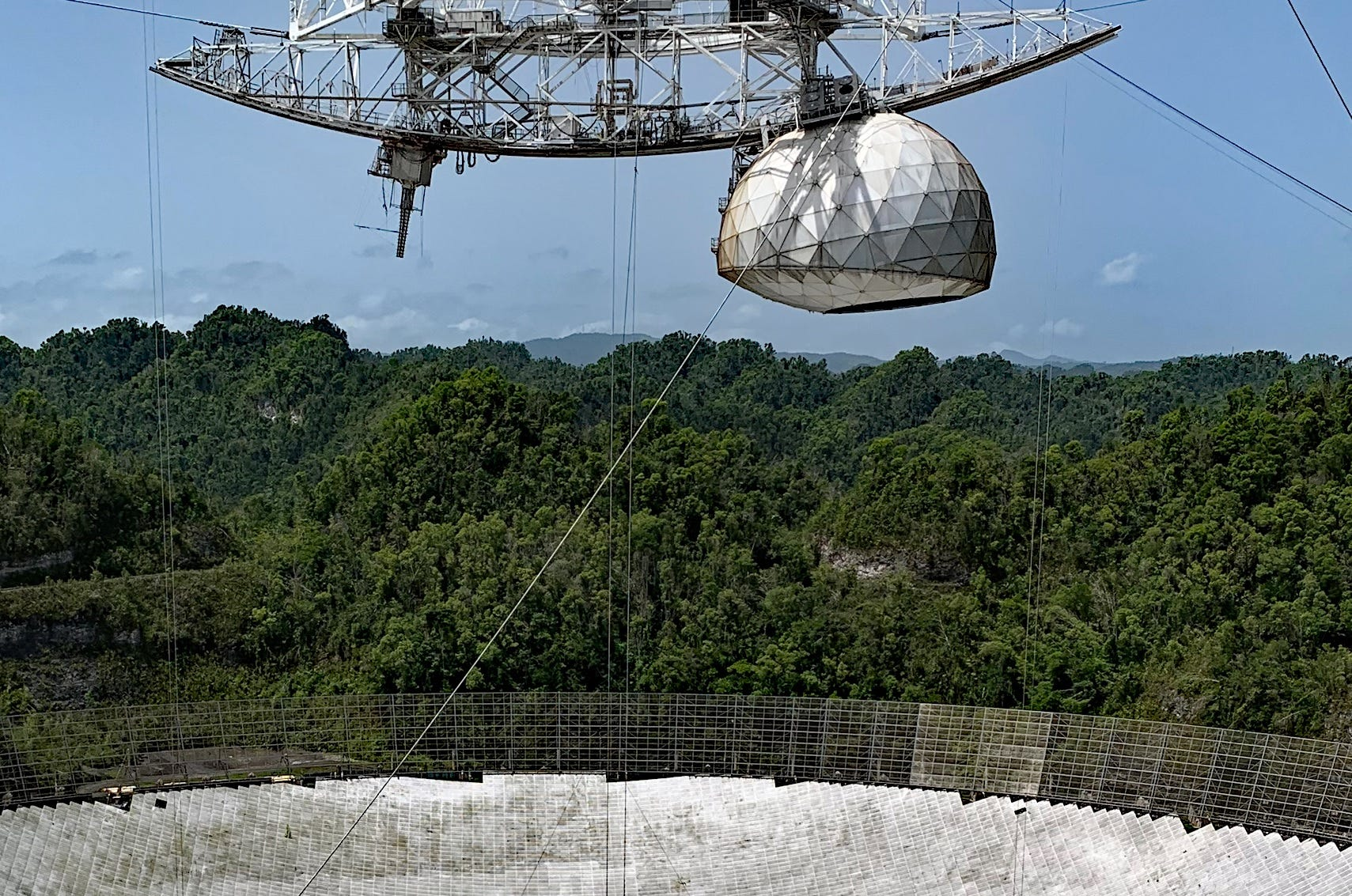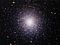# Encoding and Decoding the Arecibo 1974 SETI Message

The reason for this article is, in fact, sad. The world-famous radio telescope of the Arecibo Observatory in Puerto Rico has collapsed and is beyond repair. For many years it was the largest radio telescope in the world (diameter 304 m, frequency range up to 10 GHz), with the help of which many discoveries were made. On this Wikipedia image it is still in working condition:Source: Wikipedia

But the article is actually about another event. In 1974, a message to extraterrestrial civilizations was sent into space from this telescope. How it was encoded, let’s figure it out.

# Encoding

First, it’s interesting to understand how the message was made. As we know, the message size was only 1679 bits (approximately 210 bytes), and it was transmitted at a 2380 MHz frequency with a power of 450 kW. Frequency modulation with 10 bit/s speed was used for transmission. The number 1679 was specially chosen — it is the product of two prime numbers 23 and 73, so there is only one way to draw the picture in the form of a rectangle.

I could not find this message in WAV format, but it was easy to find it in binary form and using Python we can easily generate sound. Those wishing to listen to what the aliens will hear, can download and run the code below. Background noise has also been added to the message to make it looks more real.

`import scipy.io.wavfile as wavimport scipy.signal as signalimport numpy as npimport matplotlib.pyplot as pltfrom PIL import Imageimport osmessage = """0000001010101000000000000101000001010000000100100010001000100             1011001010101010101010100100100000000000000000000000000000000             0000011000000000000000000011010000000000000000000110100000000             0000000000101010000000000000000001111100000000000000000000000             0000000001100001110001100001100010000000000000110010000110100             0110001100001101011111011111011111011111000000000000000000000             0000010000000000000000010000000000000000000000000000100000000             0000000001111110000000000000111110000000000000000000000011000             0110000111000110001000000010000000001000011010000110001110011             0101111101111101111101111100000000000000000000000000100000011             0000000001000000000001100000000000000010000011000000000011111             1000001100000011111000000000011000000000000010000000010000000             0100000100000011000000010000000110000110000001000000000011000             1000011000000000000000110011000000000000011000100001100000000             0110000110000001000000010000001000000001000001000000011000000             0010001000000001100000000100010000000001000000010000010000000             1000000010000000100000000000011000000000110000000011000000000             1000111010110000000000010000000100000000000000100000111110000             0000000010000101110100101101100000010011100100111111101110000             1110000011011100000000010100000111011001000000101000001111110             0100000010100000110000001000001101100000000000000000000000000             0000000001110000010000000000000011101010001010101010100111000             0000001010101000000000000000010100000000000000111110000000000             0000001111111110000000000001110000000111000000000110000000000             0110000000110100000000010110000011001100000001100110000100010             1000001010001000010001001000100100010000000010001010001000000             0000001000010000100000000000010000000001000000000000001001010             00000000001111001111101001111000"""def fftnoise(f):    f = np.array(f, dtype='complex')    n_p = (len(f) - 1) // 2    phases = np.random.rand(n_p) * 2 * np.pi    phases = np.cos(phases) + 1j * np.sin(phases)    f[1:n_p+1] *= phases    f[-1:-1-n_p:-1] = np.conj(f[1:n_p+1])    return np.fft.ifft(f).realdef band_limited_noise(min_freq, max_freq, samples, samplerate):    freqs = np.abs(np.fft.fftfreq(samples, 1/samplerate))    f = np.zeros(samples)    idx = np.where(np.logical_and(freqs>=min_freq, freqs<=max_freq))    f[idx] = 1    return fftnoise(f)message = ''.join(i for i in message if i.isdigit())print("Original message:")print(message)print()# Generate messagefs = 11025f1, f2 = 3000, 4000t_sym = 0.1data = np.zeros(int(fs * t_sym * len(message)))for p in range(len(message)):    samples = np.linspace(0, t_sym, int(fs * t_sym), endpoint=False)    freq = f2 if message[p] == '1' else f1    data[int(fs * t_sym)*p:int(fs * t_sym)*(p + 1)] = 10000*(0.25*np.sin(2 * np.pi * freq * samples) + band_limited_noise(50, 5000, len(samples), fs))wav.write('arecibo.wav', fs, np.int16(data))print("WAV file saved")`

For the convenience of listening, I increased the frequency shift, in the original message it was only 10 Hz. I also placed a temporary link for those who want to listen to the WAV file without running the code.

By the way, the message was sent in 1974. To this location:Source: Wikipedia

The beautiful Hercules Globular Cluster M13 in the constellation Hercules, well known to all amateur astronomers, and can be observed even with small telescopes. The cluster is 22 thousand light-years away, so the message will go on for a long time …

We figured out the encoding and the destination, now let’s imagine that we received such a message — let’s see how it can be decoded.

# Decoding

The principle of frequency modulation itself is simple — different frequencies correspond to zero and one. On the spectrum, it looks something like this:

There are different ways to decode FSK, as the simplest method, let’s just filter one of the frequencies:

`fs, data = wav.read('arecibo.wav')def butter_bandpass(lowcut, highcut, fs, order=5):    nyq = 0.5 * fs    low = lowcut / nyq    high = highcut / nyq    b, a = signal.butter(order, [low, high], btype='band')    return b, adef butter_bandpass_filter(data, lowcut, highcut, fs, order=5):    b, a = butter_bandpass(lowcut, highcut, fs, order=order)    y = signal.lfilter(b, a, data)    return yf1, f2 = 3000, 4000data_f2 = butter_bandpass_filter(data, f2 - 200, f2 + 200, fs, order=3)plt.plot(data)plt.plot(data_f2)plt.xlabel("Time")plt.ylabel("Amplitude")plt.title("Signal")plt.show()`

The result looks good — each peak on the graph is a bit “1”:

Of course, the real signal that has passed 21 thousand years in space is likely to be “slightly” weakened, but for simplicity, I will assume that the aliens have good (and obviously not Chinese) radio receivers …

We can easily determine the width of one bit from the picture. Next, we need to output all bits as an image. Because the message was sent to an extraterrestrial civilization — those who, by definition, do not know the “earth-based” coding standards— transmitting a raster image was the only suitable decision. 21 thousand light-years from Earth, most likely, nobody knows what ASCII or Unicode is, but it is most likely possible to display the raster on the screen — anywhere in the Galaxy. At least a civilization capable of receiving a digital signal is likely to have some kind of monitor to display it.

Let’s continue the decoding. We do not know the size of the picture, but we know the size of one bit and we know the size of the entire message. Then we can just check all the possible options since there are not so many of them:

`ss = 1102  # Width of one symbol in samplesfor iw in range(12*ss, 25*ss, ss):    w, h = iw, 80    image = Image.new('RGB', (w, h))    px, py = 0, 0    for p in range(data_f2.shape):        image.putpixel((px, py), (0, int(data_f2[p]//32), 0))        px += 1        if px >= w:            px = 0            py += 1            if py >= h:                break    image = image.resize((w//10, 100*h))    image.save("1/image-%d.png" % iw)`

For clarity, the picture was stretched, because it’s hard to watch 23 pixels wide images on the modern screen. The result is clearly visible:

The final image:

Unlike images published on Wikipedia, the original image is monochrome, there is no color coding in the signal.

A lot of things are encoded in the picture (a sort of, for sure), for example, a vertical line of two pixels above a person’s head is a DNA spiral (it’s obvious, isn’t it?). The meaning of all other pictograms can be found on Wikipedia page.

# Conclusion

As we can see, quite a lot of information can be encoded into 210 bytes. In general, the task of sending a signal into deep space is far from simple, because we can use only the simplest modulation methods. Will the message reach the addressee? Of course, it’s highly unlikely. I do not know if the possibility of such a “communication line” and the required sensitivity of the receiver were evaluated. But, this is actually not so important — if such actions inspired someone to know more about space, then it was not in vain. Well, we will be able to get the answer in 44 thousand years, and I will try to update the article as soon as new data becomes available ;)

Python and IoT Developer, science and ham radio enthusiast

## More from Dmitrii Eliuseev

Python and IoT Developer, science and ham radio enthusiast